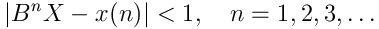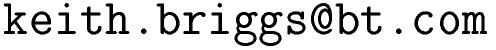Keith Briggshome · papers · thesis · talks · meetings · records · maths notes · software « · languages · music · travel · cv · students · maps · place-names · people · photos · links · ex libris · site map### xrc (exact reals in C)

This is an implementation of lazy exact real arithmetic in C. It is an alternative to multiple-precision floating-point codes. An important distinction is that in multiple-precision floating-point, one sets the precision before starting a computation, and then one cannot be sure of the final result. Interval arithmetic is an improvement on this, but still not an ideal solution because if the final interval is larger than desired, there is no simple way to restart the computation at higher precision. By constrast, in exact real arithmetic no precision level is set in advance, and no computation takes place until a final request takes place for some output. I have previously developed similar codes in python and C++. This new C version is faster, more portable, and easier to use.

#### usage

See the man page.

#### how does it work?

In this code, to each (mathematical) real number X in R there corresponds an xr_t x, which is a function int -> mpz_t, where mpz_t is an arbitrary precision integer type. This function is normally not called by the user. It always satisfieswhere X is the real represented by x. This inequality is propagated through all operations.

B=2b is typically 2=21. b can be chosen by the user (1,2,3,...), but 1 seems a good value. All results should be invariant with respect to b.

#### applications

The intended applications are proving inequalities between constructed reals. I initially developed this code as I had a need in my work on simultaneous Diophantine approximation algorithms. A typical situation there is that we have two irrational numbers a1,a2 and some very large integers p1,p2,q such that e1=|q*a1-p1| and e2=|q*a2-p2| are very small and very close, but we have to decide which is smaller. The result of such a computation with xrc is guaranteed correct.

A simpler well-known example is this: suppose we want to know whether exp(p*sqrt(163)) is an integer or not. In double precision, we cannot conclude anything:

double x=exp(3.141592653589793*sqrt(163));
cout << setprecision(16) << x; // prints 262537412640767947.

but in xrc we can just do this:

x=xr_exp(xr_pi()*xr_sqrt(xr_init(163,1)));
y=xr_nearint(x);
printf("%d",y!=x);

which is a proof that x is not an integer, since the output means that it is strictly greater than its floor and strictly less than its ceiling.

There are probably other applications in computational geometry, but this is NOT the right code to use if you want to look at a million decimals of p!

#### other attempts at similar things

Some possibly competing projects with xrc include:

#### webliography

I obtained theoretical ideas and algorithms from these sites:

Some of the earlier work on exact reals used continued fraction arithmetic:
Gosper's famous continued fraction arithmetic hakmem and the less well-known but just as important second part.
J. Vuillemin: `Exact real computer arithmetic with continued fractions', INRIA Rapports de Recherche 760 (1987); ACM Symposium on lisp and functional programming 1988, pp. 14-27; and IEEE Transactions on Computers 39, 1087-1105, 1990.
P. Liardet and P. Stambul: Algebraic Computations with Continued Fractions.

This website uses no cookies. This page was last modified 2013-05-12 10:17 by.